# Characterization and Boronizing Kinetics of EN-GJL-250 Lamellar Gray Cast Iron

Characterization and Boronizing Kinetics of EN-GJL-250 Lamellar Gray Cast Iron

Laboratory of Materials Technology, Faculty of Mechanical and Process Engineering, University of Sciences and Technology Houari Boumediene, Bab-Ezzouar, Algiers, Algeria

Institute of Materials Science and Engineering, Poznan University of Technology, Poznan, Poland

Corresponding Author Email:
mkeddam@usthb.dz
Page:
23-28
|
DOI:
https://doi.org/10.18280/acsm.440103
18 August 2019
|
Accepted:
5 November 2019
|
Published:
15 March 2020
| Citation

OPEN ACCESS

Abstract:

The aim of the present study is to generate the boride coatings at the surface of the lamellar gray cast iron (EN-GJL-250). This material was hardened by pack-boriding in the powders mixture of 50% B4C, 49.5% Al2O3 and 0.5% AlF3 at 800, 900 and 1000°C for 4, 6 and 8 h. The produced borided layers were characterized by Scanning electron microscopy (SEM) and XRD analysis. The boriding kinetics of EN-GJL-250 lamellar gray cast iron was also investigated. Based on the experimental data, the value of activation energy for boron diffusion was calculated as 163.86 kJ mol-1 for the EN-GJL-250 cast iron by using the integral diffusion model. The regression model based on the design of experiments (DOE) was also employed in order to predict the total boride layer thickness as a function of boriding parameters (the treatment time and the boriding temperature). The experimental values of total boride layer thickness were in agreement with the results from the regression model.

Keywords:

pack-boriding, kinetics, activation energy, design of experiments, integral diffusion model

1. Introduction

The boriding process is a thermochemical treatment applied to produce a hard layer composed of borides on ferrous and non-ferrous alloys. In case of ferrous alloys (steels and cast irons), two kinds of iron borides can be formed depending on the chemical composition of boriding agent and on the process parameters (the time duration and the boriding temperature). As a result of this thermochemical process, the surface properties and wear performance of treated parts are improved due to a high hardness of boride layers . The boriding process requires high temperatures to be carried out (between 800 and 1050°C) and a treatment time from 0.5 to 10 h. Various methods of boriding can be found in the literature. Among them, the powder pack-boriding as the frequently used method, owing to its simplicity and cost effectiveness [2, 3]. For instance, Sen et al.  have used the liquid boriding (in salts bath) to produce the boride layers on GGG-80 ductile cast iron and investigate the wear behaviour.

Nodular cast iron 500-7 was laser borided by a CO2 molecular laser with a continuous wave and using amorphous boron as an alloying element . In the literature, a few information about the growth kinetics of gray and ductile cast irons exists. In this context, Campos-Silva et al.  have pack-borided the gray cast iron material between 1173 and 1273 K to form a single boride layer (Fe2B). They applied a diffusion model to estimate the boron diffusion coefficients in the Fe2B layers formed on gray cast iron. Mu et al.  have hardened a gray cast iron by powder pack-boriding in the temperature range 1123-1223 K to get a single Fe2B layer using a mixture of powders composed of 88% SiC, 8% B4C, and 4% KBF4.

Azouani et al.  have treated the EN-GJL-250 gray cast iron in the temperature range 800-1000°C with a mixture of powders containing 90% SiC, 5% B4C, and 5% NaBF4 to get a double boride layer (FeB+Fe2B). In this paper, a kinetic analysis was done by using the design of experiments approach. Sen et al.  have borided in salts bath three types of ductile cast irons at 850 and 950°C for 2 to 8 h. They analyzed the growth kinetics of formed boride layers at 850 and 950°C. Sen et al.  have borided a ductile cast iron in salts bath and have studied its boriding kinetics. Azouani et al.  have analyzed the boriding kinetics of EN-GJS-400-15 ductile cast iron by using the mixture of powders composed of 90% SiC, 5% B4C, and 5% NaBF4

In the current work, the EN-GJL-250 lamellar gray cast iron was hardened by the powder-pack boriding in the temperature range 800-1000°C for a treatment time of 4-8 h.

The morphology of produced boride layers was observed by SEM. The crystallographic nature of iron borides was identified by XRD analysis. Furthermore, the activation energy for boron diffusion in EN-GJL-250 lamellar gray cast iron was estimated by using the integral diffusion model and a comparison was made with the literature results.

A comparison of experimental total boride layers’ thicknesses with the simulated values from the regression model was finally done.

The present research paper is organized in 5 sections. Section 1 presents an overview of existing research background and significance of the studied problem. The mathematical formulation of the integral diffusion model is addressed in Section 2.

The details regarding the material and experimental procedure are given in Section 3. The estimated value of boron activation energy was compared to the data taken from the literature in Section 4. A conclusion gathering the main results was given in the last Section.

2. The Integral Diffusion Model

This kinetic approach, based on the integral diffusion model, Türkmen et al.  was adopted to be applied for the growth kinetics of entire boride layer (FeB+Fe2B) formed on the lamellar gray cast iron substrate. The integral diffusion model has been successfully used to simulate the kinetics of Fe2B layers on different substrates [12-18]. The developed boron concentration-profile through the entire boride layer is schematically shown in Figure 1.

## 1.png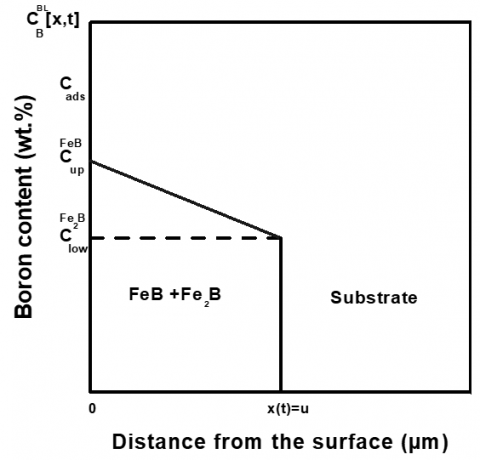Figure 1. A schematic illustration of boron-concentration profile through the entire boride layer

CupFeBdenotes the upper limit of boron concentration in the FeB phase (=16.40 wt.%) while represents the lower limit of boron concentration in the Fe2B phase (=8.83 wt.%) and the variable u represents the entire boride layer thickness (FeB+Fe2B). The term Cads is the adsorbed boron concentration in the entire boride layer during the boriding treatment . C0 is the boron solubility in the matrix which can be neglected because of its very low concentration ($\approx$0 wt.%) .

The assumptions considered during the formulation of this diffusion model were given elsewhere [12-18]. The initial and boundary conditions for this diffusion problem are represented as:

$t=0, x>0,$ with $C_{B}^{B L}[x(t), t=0]=C_{0} \approx 0$ wt.%B   (1)

Boundary conditions:

$C_{B}^{B L}[x(t=0)=0, t=0]=C_{u p}^{F e B}$

for $C_{a d s}>16.23 \mathrm{wt.} \% \mathrm{B}$     (2)

$C_{B}^{B L}[x(t=t)=u(t), t=t]=C_{l o w}^{F e_{2} B}$

for $C_{a d s}<8.83 \mathrm{wt} . \% \mathrm{B}$    (3)

The change in the boron concentration inside the entire boride layer (FeB+Fe2B) as a function of the distance x(t) at a given boriding temperature is described by the Second Fick’s law:

$D_{B}^{B L} \frac{\partial^{2} C_{B}^{B L}[x, t]}{\partial x^{2}}=\frac{\partial C_{B}^{B L}[x, t]}{\partial t}$   (4)

where, DBBL  is the boron diffusion coefficient in the entire boride layer (FeB+Fe2B). The expression of the boron-concentration profile along the entire boride layer was taken from the Goodman’s method  as follows:

$C_{B}^{B L}[x, t]=C_{l o w}^{F e_{2} B}+a(t)[u(t)-x]+b(t)[u(t)-x]^{2}$ for $0 \leq x \leq u$    (5)

The three time-dependent unknowns a(t), b(t) and u(t) are subjected to the boundary conditions given by Eqns. (2) and (3). Eq. (6), which is the first algebraic constraint, was obtained by considering the boundary condition on the surface:

$a(t) u(t)+b(t) u(t)^{2}=\left(C_{u p}^{F e B}-C_{l o w}^{F e_{2} B}\right)$    (6)

The ordinary differential equation (ODE) given by Eq. (7) was Clow​Fe2Bobtained after integration of Eq. (4) with respect to the diffusion distance x(t) from 0 to u(t):

$\frac{d}{d t}\left[\frac{u(t)^{2}}{2} \frac{d a(t)}{d t}+\frac{u(t)^{3}}{3} \frac{d b(t)}{d t}\right]=2 D_{B}^{B L} b(t) u(t)$   (7)

The second algebraic constraint was derived from the mass balance equation at the (FeB+Fe2B/substrate) interface:

$\left(C_{u p}^{F e B}+C_{l o v}^{F e_{2} B}\right) b(t)=a(t)^{2}$   (8)

Eqns. (6), (7) and (8) represent a system of differential algebraic equations (DAE) whose unknowns are the variables a(t), b(t) and u(t). By considering the analytic solution of this diffusion problem, the expression of boron diffusion coefficient in the total boride layer (FeB+Fe2B) was deduced as follows (Table 1):

$D_{B}^{B L}=\eta k^{2}$    (9)

With:

$\left.\eta=\left[\left(\frac{1}{16}\right)\left(\frac{C_{u p}^{F e B}+C_{l o w}^{F e_{2} B}}{C_{u p}^{F e B}-C_{l o w}^{F e_{2} B}}\right)\left(1+\sqrt{1+4\left(\frac{C_{u p}^{F e B}-C_{l o v}^{F e_{2} B}}{C_{u p}^{F e B}+C_{l o w}^{F e_{2} B}}\right)}\right.\right)+\left(\frac{1}{12}\right)\right]$

k represents the parabolic growth constant at the (FeB+Fe2B /substrate) interface or the slope of the straight line relating the entire boride layer thickness u(t) to the square root of time.

$u(t)=k \sqrt{t}$    (10)

The η constant was found to be equal to 0.60061. The expression of entire boride layer thickness from the integral method can be finally re-written as follows:

$u(t)=\sqrt{\frac{D_{B}^{B L}}{\eta} t}$    (11)

Table 1. The experimentally determined parabolic growth constants and the calculated values of boron diffusion coefficients by using the integral diffusion model (Eq. (9))

 T (°C) k (µm s -0.5) DBBL(µm2s-1) 800 0.27009 0.04381 900 0.60792 0.22197 1000 1.1413 0.78239
3. Experimental Procedure

3.1 The material and the boriding treatment

The substrate to be hardened by the powder-pack boriding was the EN-GJL-250 lamellar gray cast iron whose chemical composition is displayed in Table 2.

Table 2. Chemical composition of EN-GJL-250 lamellar Gray cast iron (given in weight percent)

 C Si Mn P S Fe 3.1-3.4 1.9-2.3 0.6-0.9 0.05 0.02 Bal.

The samples to be borided were cut from an ingot in cubic form with dimensions of 10x10x10 mm3. The SiC abrasive papers of different granulometry (of 180 to 400) were used for grinding. They were finally cleaned with acetone to remove any residue of grease and dirt. The pack-boriding process was realized in a sealed stainless steel container placed in an electrical resistance furnace. The container was filled with the powders mixture composed of 50 wt.% B4C, 49.5 wt.% Al2O3 and 0.5 wt.%AlF3. The samples to be borided were placed in contact with the powders mixture. The process temperatures were 800, 900 and 1000°C for treatment times of 4, 6 and 8h. Once finished, the borided samples were removed from the furnace and cooled in air.

3.2 Characterization of the borided samples

The SEM observations were performed on polished and etched cross-sections of pack-borided samples using TESCAN VEGA 5135 scanning electron microscope. The measurements of boride layer thickness were made by employing a practical procedure proposed by Kunst and Schaaber  along the cross-sections of borided samples. A minimum of 20 measurements was taken to ensure a good reproducibility. The formation of iron borides at the surface of borided samples was confirmed by using an X-ray diffractometer (PHILIPS X’PERT PRO MPD) with a Cu-Ka radiation source with a wavelength of 0.154 nm. To determine the crystalline nature of phases from the diffraction peaks, the data taken from the JCPDS database were used .

4. Results and Discussions

4.1 SEM observations of the boride layers

Figure 2 shows the SEM image of the initial microstructure of EN-GL-250 lamellar cast iron after etching with 2% Nital solution. The lamellar graphite is seen inside a fully pearlitic matrix.

## 2.pngFigure 2. SEM micrograph showing the initial microstructure of EN-GJL-250 lamellar Gray cast iron

## 3a.png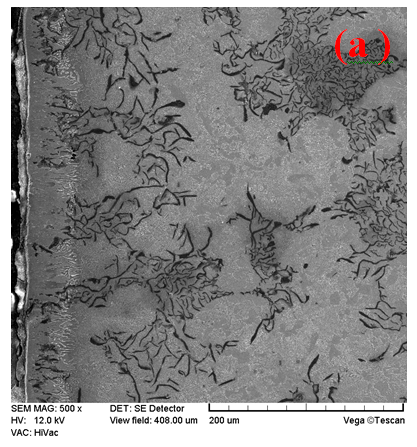## 3b.png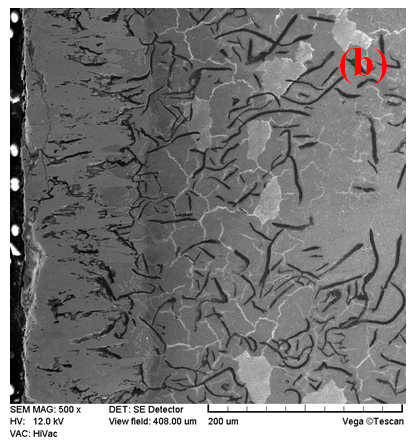## 3c.png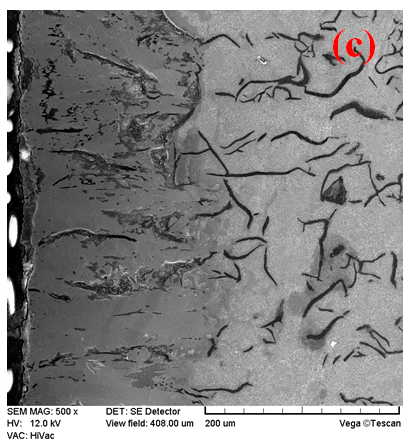Figure 3. SEM images of the cross-sections of borided samples of EN-GJL-250 lamellar gray cast iron for 8 h at different temperatures: (a) 800°C, (b) 900°C and (c) 1000°C

Figure 3 shows the cross-sections of boride layers formed on EN-GJL-250 lamellar gray cast irons at 800, 900 and 1000°C during 8 h of treatment after etching with 2% Nital solution. The boride layers exhibited a less pronounced saw-tooth morphology because of the presence of alloying elements with high contents compared to borided low alloy steels, carbon steels and Armco iron. The formation of borides needles was ascribed to the preferred pathway for boron diffusion along the crystallographic direction [0 0 1] . Furthermore, the thickness of boride layers was increased with the rise of boriding temperature since the diffusion phenomenon of boron atoms at the surface of treated material is thermally activated. The boride layer thickness reached a value of 190.39±8.3 µm at 1000°C while it is only 49.19±3 µm at 800°C for a treatment time of 8 h. No contrast could be distinguished between the both layers (FeB and Fe2B) in the SEM images since the volume fraction of FeB phase is very low. It can be concluded that the Fe2B phase is dominant in the formed boride layers whatever the boriding conditions.

4.2 XRD analysis

## 4a.png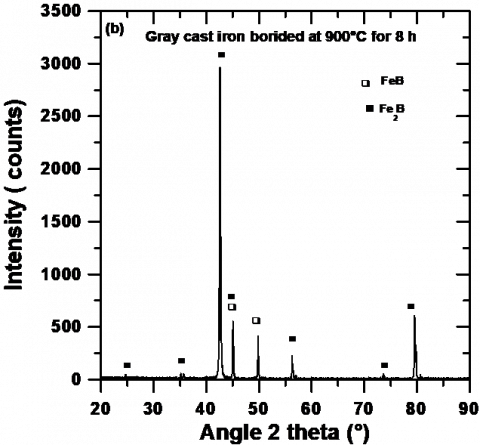## 4b.png## 4c.png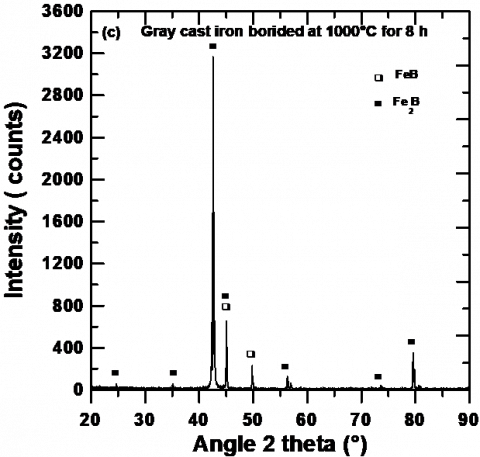Figure 4. XRD patterns recorded at the surfaces of pack-borided EN-GJL-250 lamellar gray cast iron for 8 h of treatment at the three temperatures: (a) 800°C, (b) 900°C and (c) 1000°C

Figure 4 represents the XRD patterns obtained at the surfaces of borided EN-GJL-250 lamellar cast iron for the boriding conditions (800, 900 and 1000°C for 8 h of treatment).

The presence of iron borides was verified by use of JCPDS database . Two kinds of iron borides were identified as FeB and Fe2B at the surface of treated lamellar gray cast iron. The same phases were also found for all boriding conditions. It is seen that the Fe2B phase has the strongest peak corresponding to the crystallographic plane (002) whatever the boriding temperature. The boride layer is composed mainly of Fe2B phase with a minor presence of FeB phase based on the XRD results.

4.3 Estimation of the value of activation for boron diffusion

The boriding kinetics is controlled by the diffusion rate of boron atoms inside the substrate. Therefore, the values of parabolic growth constants can be obtained from a linear fitting of the experimental entire boride layer thickness u(t) versus the square root of time according to Eq. (10). The variation of total boride layer as a function of square root of time is depicted in Figure 5 for different boriding temperatures.

## 5.png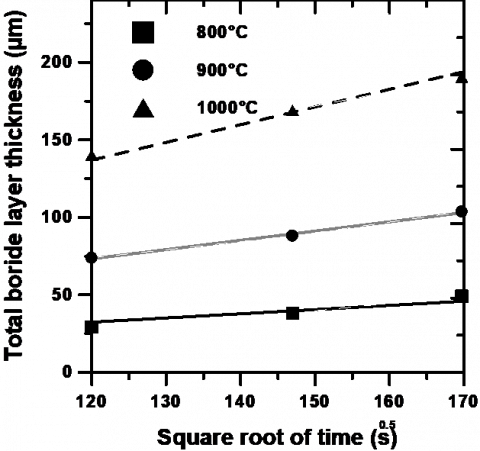Figure 5. Time dependence of the square of total boride layer thickness at different process temperatures

The parabolic character regarding the growth kinetics of boride layers is confirmed. The relationship describing the evolution of boron diffusion coefficient with the boriding temperature can be expressed by Eq. (12):

$D_{B}^{B L}=D_{0} \exp \left(-\frac{Q}{R T}\right)$   (12)

where, D0 is the diffusion coefficient of boron extrapolated at a value of (1/T) = 0. The Q parameter represents the value of activation energy for boron diffusion which gives the quantity of energy (in kJ mol-1) necessary for the reaction to occur, and R is the ideal gas constant (R=8.314 J mol-1. K-1). Figure 6 describes the evolution of natural logarithm of the calculated values of boron diffusion coefficients through the entire boride layer as a function of reciprocal temperature.

The value of activation energy for boron diffusion Q can be readily obtained from the slope of the curve relating the natural logarithm of $D_{B}^{B L}$ to the inverse of temperature. Eq. (13) was obtained from the graph displayed in Figure 6 by a linear fitting of data with a coefficient of determination close to unity.

$D_{B}^{B L}=4.2317 \times 10^{-6} \exp \left(-\frac{163.86 \mathrm{kJ} / \mathrm{mol}}{R T}\right)$    (13)

Table 3. Values of activation energies for boron diffusion in the pack-borided cast irons

 Material Q (kJ mol-1) References ASTM A-536 197.28  Gray cast iron 177.4  Gray cast iron 209.0  EN-GJS-400-15 212.28  ASTM A-536 155.7  EN-GJL-200 163.86 This work

In Table 3 are gathered the values of activation energies for boron diffusion in some pack-borided cast irons [3, 6, 11, 25] with the value of activation energy for boron diffusion in EN-GJL- 250 lamellar gray cast iron estimated in this work. The found value of activation energy for boron diffusion (=163.86 kJ mol-1) is required to overcome the energetic barrier allowing the diffusion of boron atoms inside the material substrate.

## 6.png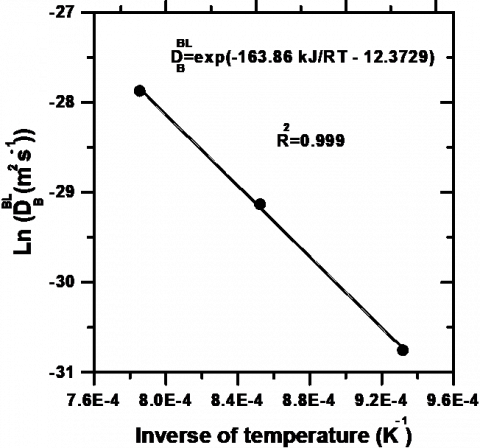Figure 6. Arrhenius relationship between the boron diffusion coefficient and the process temperature

4.4 Prediction of total boride layer thickness with the regression model

A full factorial design with two factors at three levels was used to predict the total boride layers’ thicknesses depending upon the boriding parameters (time and temperature) by means of ANOVA analysis [26, 27]. Based on the experimental data, Equation (14) was obtained as follows:

$u(T, t)=816.31167-2.14797 \times T-23.88917 \times t+0.03812 \times T \times t+0.00142 \times T^{2}-0.17500 \times t^{2}$

for 800<T<1000°C and 4<t<8 h   (14)

where, t represents the time duration (h) and T the process temperature in degree Celsius.

Equation (14) can be used to get the iso-thickness diagram shown in Figure 7.

This Figure allows selecting the optimum value of total boride layer thickness according to the practical utilization of EN-GJL-250 lamellar gray cast iron.

Table 4 provides a comparison between the experimental values of total boride layers’ thicknesses and the predicted values using Equation (14) in the temperature range 800-1000°C. It is noticed that the predicted values of total boride layers’ thicknesses agree with the experimental results.

## 7.png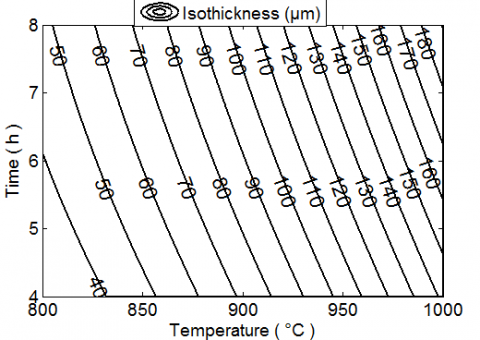Figure 7. Iso-thickness diagram showing the variation of total boride layer thickness as a function of the boriding parameters

Table 4. Comparison between the experimental values of total boride layers’ thicknesses and those provided by regression model (Equation (14)) in the temperature range 800-1000°C

 Total boride layer thickness (µm) T (°C) t (h) Experimental values Simulated values 800 4 29.39±1.6 30.36 6 38.29±3.4 40.07 8 49.19±3.0 48.39 900 4 73.85±6.2 72.24 6 88.08±7.1 89.55 8 103.63±7.9 105.48 1000 4 140.1±7.6 142.46 6 169.0±7.2 167.42 8 190.39±8.4 190.98
5. Conclusions

In this work, the substrates made of EN-GJL-250 lamellar gray cast iron were pack-borided in the temperature range 800-1000°C between 4 and 8 h. The formed boride layers on EN-GJL-250 lamellar gray cast iron were composed of FeB and Fe2B phases with a less pronounced saw-tooth morphology. The XRD results indicated that Fe2B is the dominant phase in the boride layer in accordance with the SEM observations. The total boride layer thickness attained a value of 190.39± 8.4 µm during 8 h for 1000°C, while it was only 29.39±1.6 µm for 4 h at 800°C.

Based on the integral diffusion model, the value of activation energy for boron diffusion in EN-GJL-250 lamellar gray cast iron was found to be 163.86 kJ mol-1 and compared with the values of energy available in the literature. Furthermore, an iso-thickness diagram yielding the total boride layer thickness (FeB+ Fe2B) as a function of boriding parameters (time and temperature) was suggested based on the design of experiments. It could be used as a simple tool to select the optimum values of total boride layer thickness for a practical use of EN-GJL-250 lamellar gray cast iron in the industry. Finally, the experimental values of total boride layer thickness were in agreement with the values given by the regression model.

As a prospect, this applied integral diffusion model can be used to investigate the boriding kinetics of any borided ferrous alloys.

References

 Sinha, A.K. (1991). ASM Handbook - Heat Treating. ASM International, Vol. 4: 437-446.

 Keddam, M., Chentouf, S.M. (2005). A diffusion model for describing the bilayer growth (FeB/Fe2B) during the iron powder-pack boriding. Applied Surface. Science, 252(2): 393-399. https://doi.org/10.1016/j.apsusc.2005.01.016

 Keddam M. (2018). A diffusion model for the Fe2B layers formed on a ductile cast iron. Acta Physica Polonica A, 133: 1174-1177. https://doi.org/10.12693/APhysPolA.133.1174

 Sen, U., Sen, S. Yilmaz, F. (2005). Effect of process time on the tribological properties of boronized GGG-80 ductile cast iron. Industrial Lubrication and Tribology, 57(6): 243-248. https://doi.org/10.1108/00368790510622335

 Paczkowska, M., Ratuszek, W., Waligora, W. (2010). Microstructure of laser boronized nodular iron. Surface and Coatings Technology, 205(7): 2542-2545. https://doi.org/10.1016/j.surfcoat.2010.09.057

 Campos-Silva, I., Lopez-Perrusquia, N., Ortiz-Dominguez, M., Figueroa-Lopez, U., Gomez-Vargas, O.A., Meneses-Amador, A., Rodriguez-Castro, G. (2009). Characterization of boride layers formed at the surface of gray cast irons. Kovove Mater, 47(2): 75-81.

 Mu, D., Shen, B.L. (2013). The kinetics and dry-sliding wear properties of boronized gray cast iron. Advances in Materials Science and Engineering, 2013: 1-7. http://dx.doi.org/10.1155/2013/751971

 Azouani, O., Keddam, M., Allaoui, O., Sehisseh, A. (2017). Kinetics of formation of boride layers on EN-GJL-250 gray cast iron. Materials Performance and Characterization, 6: 510-522. https://doi.org/10.1520/MPC20160077

 Sen, U., Sen, S., Yilmaz, F. (2004). Improving the surface properties of ductile irons by boronizing. Key Engineering Materials, 264-268: 541-544. https://doi.org/10.4028/www.scientific.net/KEM.264-268.529

 Sen, U., Sen, S., Yilmaz, F., (2004). An evaluation of some properties of borides deposited on boronized ductile iron. Journal of Materials Processing Technology, 148: 1-7. https://doi.org/10.1016/j.jmatprotec.2004.01.015

 Azouani, O., Keddam, M., Allaoui, O., Sehisseh, A. (2017). Characterization of boride coatings on a ductile cast iron. Protection of Metals and Physical Chemistry of Surfaces, 53: 306-311. https://doi.org/10.1134/S207020511702006X

 Türkmen, I., Yalamaç, E., Keddam, M. (2019). Investigation of tribological behaviour and diffusion model of Fe2B layer formed by the pack-boriding process on SAE 1020 steel. Surface and Coatings Technology, 377: 124888. https://doi.org/10.1016/j.surfcoat.2019.08.017

 Ortiz-Domínguez, M., Keddam, M., Elias-Espinosa, M., Ramírez-Cardona, M., Arenas-Flores, A., Zuno-Silva, J., Cervantes-Sodi, F., Cardoso-Legorreta, E. (2019). Characterization and boriding kinetics of AISI T1 steel. Metall. Res. Technol, 116: 102. https://doi.org/10.1051/metal/2018081

 Elias-Espinosa, M., Keddam, M., Ortiz-Domínguez, M., Arenas-Flores, A., Zuno-Silva, J., Cervantes-Sodi, F., Reyes-Retana, J.A. (2019). Investigation of growth kinetics of Fe2B layers on AISI 1518 steel by the integral method. High Temperature Materials and Processes, 38: 219-228. https://doi.org/10.1515/htmp-2017-0166

 Keddam, M., Ortiz-Dominguez, M., Elias-Espinosa, M., Arenas-flores, A., Zuno-Silva, J., Zamarripa-Zepeda, D., Gomez-Vargas, O.A. (2018). Kinetic investigation and wear properties of Fe2B layers on AISI 12L14 steel. Metallurgical and Materials Transactions A, 49: 1895-1907. https://doi.org/10.1007/s11661-018-4535-1

 Keddam, M., Kulka, M. (2018). Analysis of the growth kinetics of Fe2B layers by the integral method. J. Min. Metall. Sect. B-Metall, 54(3): 361-367. https://doi.org/10.2298/JMMB180405026K

 Zuno, J., Keddam, M., Ortiz-Domínguez, M., Elias-Espinosa, M., Cervantes-Sodi, F., Oseguera-Peña, J., Fernández De-Dios, L.D., Gómez-Vargas, O.A. (2018). Kinetics of formation of Fe2B layers on AISI S1 steel. Materials Research, 21(5): e20180173. http://dx.doi.org/10.1590/1980-5373-mr-2018-0173

 Keddam, M., Elias-Espinosa, M., Ortiz-Domínguez, M., Simón-Marmolejo, I., Zuno-Silva, J. (2017). Pack-boriding of AISI P20 steel: Estimation of boron diffusion coefficients in the Fe2B layers and tribological behavior. International Journal of Surface Science and Engineering, 11(6):  563-585. http://dx.doi.org/10.1504/IJSURFSE.2017.088997

 Yu, L.G., Chen, X.J., Khor, K.A., Sundararajan, G. (2005). FeB/Fe2B phase transformation during SPS pack-boriding: Boride layer growth kinetics. Acta Materialia, 53(8): 2361-2368. https://doi.org/10.1016/j.actamat.2005.01.043

 Okamoto, H. (2004). B-Fe (boron-iron). Journal of Phase Equilibria and Diffusion, 25(3): 297-298. https://doi.org/10.1007/s11669-004-0128-3

 Goodman, T.R. (1964). Application of integral methods to transient nonlinear heat transfer. Adv. Heat Transfer, 1: 51-122. https://doi.org/10.1016/S0065-2717(08)70097-2

 Kunst, H., Schaaber, O. (1976). Beobachtungen beim Oberflaechenborieren von Stahl II. Harterei Tech. Mittenlungen, 22: 275-292.

 JCPDS- International Centre for Diffraction Data, PCPDF WIN, Version 202 (1999).

 Brakman, C.M., Gommers, A.W.J., Mittemeijer, E.J. (1989). Boriding of Fe and Fe–C, Fe–Cr, and Fe–Ni alloys; Boride-layer growth kinetics. J. Mater. Res., 4(6): 1354-1370. https://doi.org/10.1557/JMR.1989.1354

 Perrusquia, N., Donu Ruiz, M.A., Vargas, E.Y.O., Suarez V.C. (2012). Diffusion of hard coatings on ductile cast iron. Mater. Res. Soc. Symp. Proc., 1481: 105-112. https://doi.org/10.1557/opl.2012.1638

 Goupy, J. (2001). Plans d’expériences pour surfaces de réponses, Edition Dunod.

 Daryl, S. (2007). Paulson, Handbook of regression and modeling, Chapman & Hall/CRC Biostatistics Series, New York.